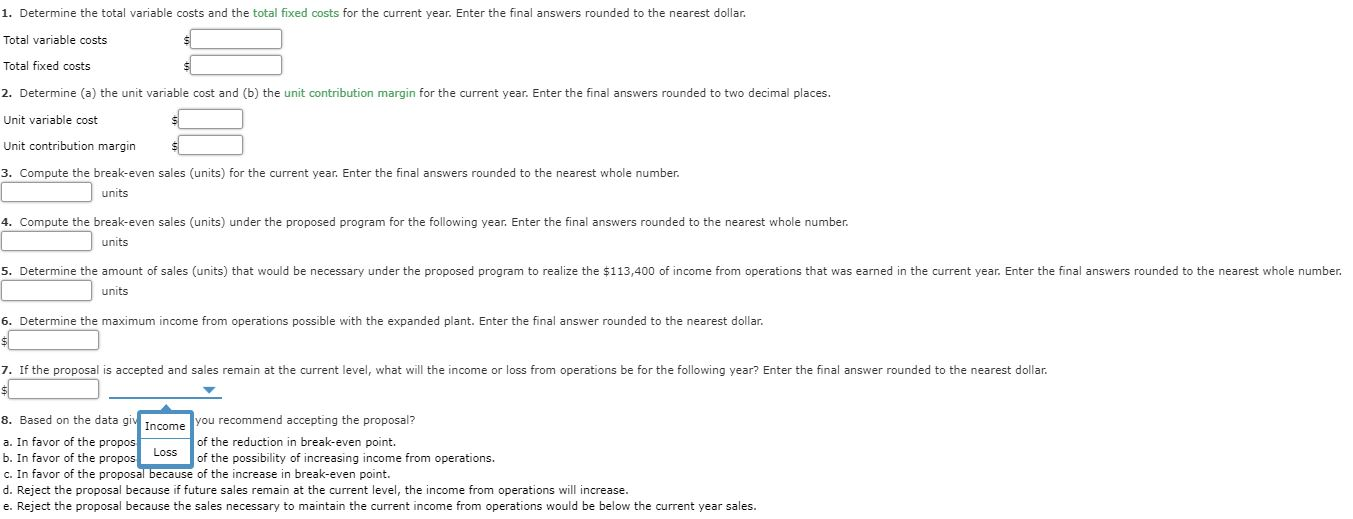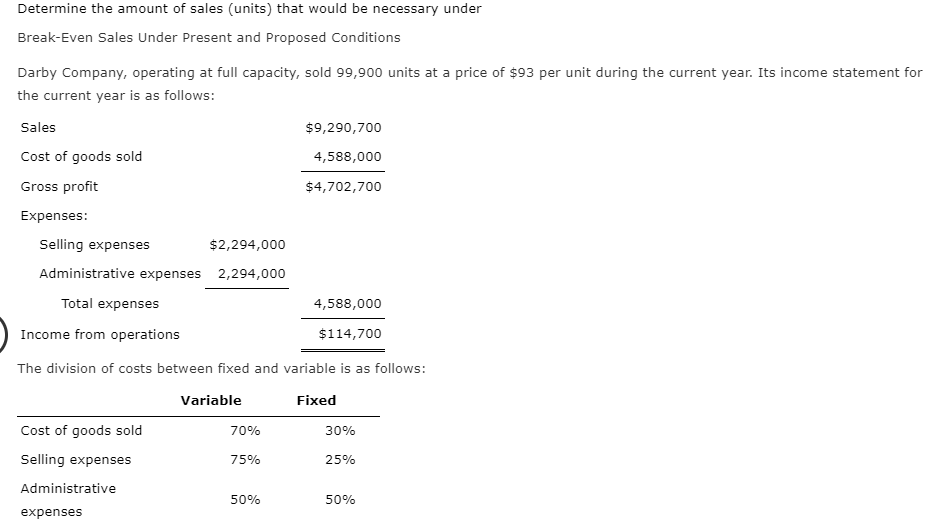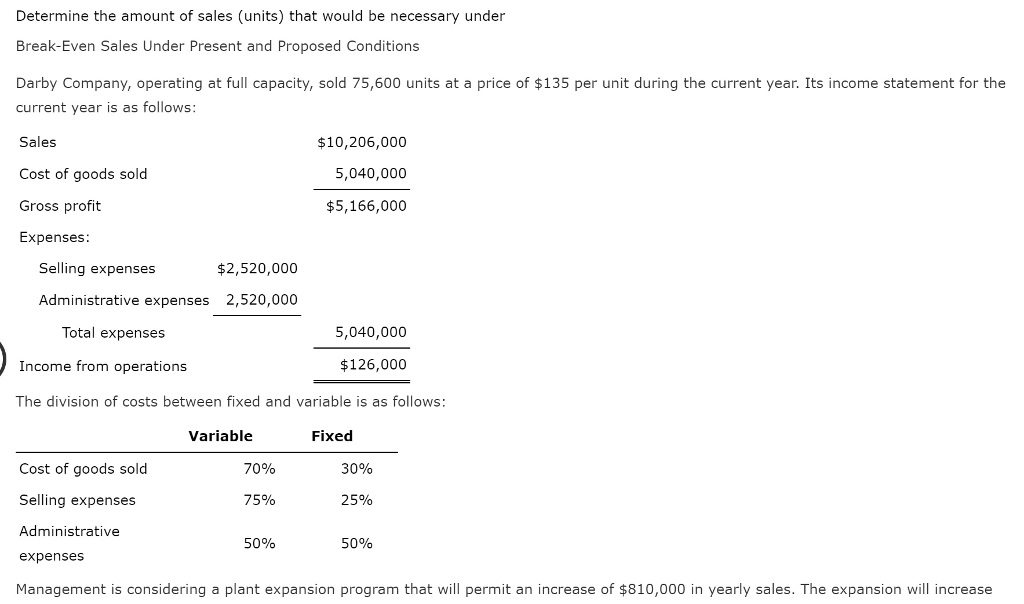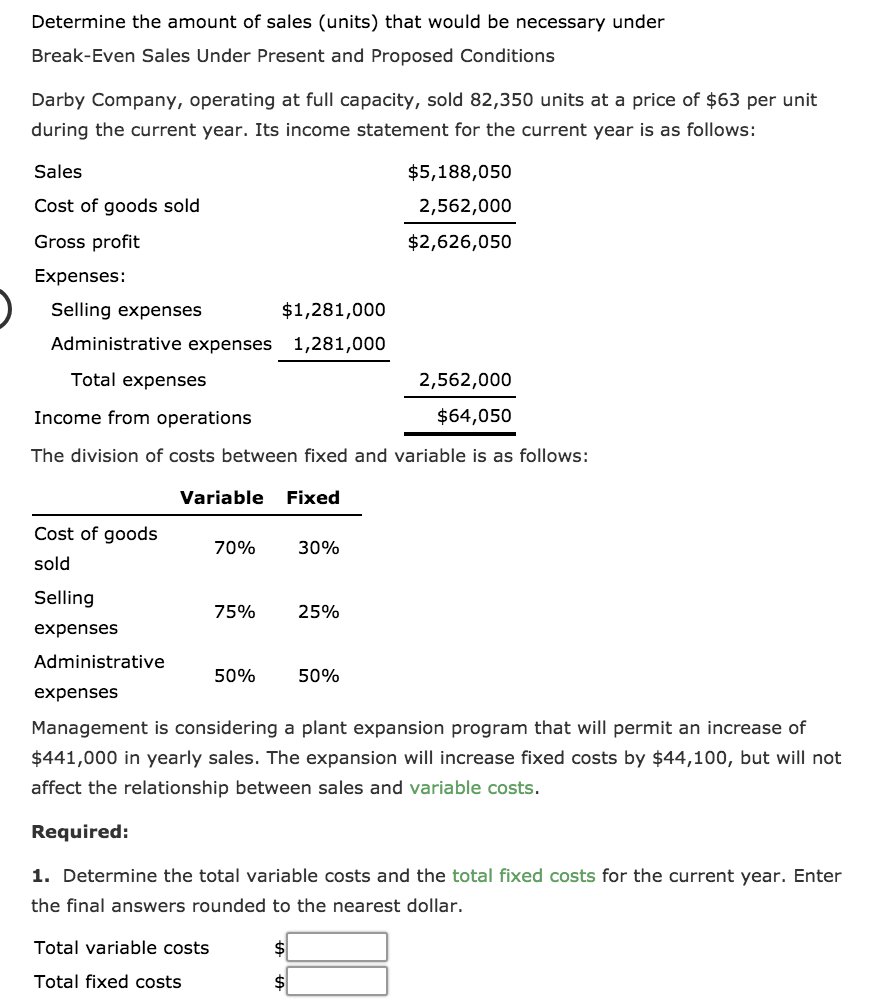# Determine the amount of sales (units) that would be necessary under Break-Even Sales Under Present and...

Determine the amount of sales (units) that would be necessary under
Break-Even Sales Under Present and Proposed Conditions

Darby Company, operating at full capacity, sold 72,900 units at a price of \$135 per unit during the current year. Its income statement for the current year is as follows:

Sales \$9,841,500
Cost of goods sold 4,860,000
Gross profit \$4,981,500
Expenses:
Selling expenses \$2,430,000
Total expenses 4,860,000
Income from operations \$121,500
The division of costs between fixed and variable is as follows:

Variable Fixed
Cost of goods sold 70% 30%
Selling expenses 75% 25%
Management is considering a plant expansion program that will permit an increase of \$945,000 in yearly sales. The expansion will increase fixed costs by \$94,500, but will not affect the relationship between sales and variable costs.

Required:

1. Determine the total variable costs and the total fixed costs for the current year. Enter the final answers rounded to the nearest dollar.

Total variable costs \$
Total fixed costs \$
2. Determine (a) the unit variable cost and (b) the unit contribution margin for the current year. Enter the final answers rounded to two decimal places.

Unit variable cost \$
Unit contribution margin \$
3. Compute the break-even sales (units) for the current year. Enter the final answers rounded to the nearest whole number.
units

4. Compute the break-even sales (units) under the proposed program for the following year. Enter the final answers rounded to the nearest whole number.
units

5. Determine the amount of sales (units) that would be necessary under the proposed program to realize the \$121,500 of income from operations that was earned in the current year. Enter the final answers rounded to the nearest whole number.
units

6. Determine the maximum income from operations possible with the expanded plant. Enter the final answer rounded to the nearest dollar.
\$
7. If the proposal is accepted and sales remain at the current level, what will the income or loss from operations be for the following year? Enter the final answer rounded to the nearest dollar.
\$

8. Based on the data given, would you recommend accepting the proposal?

In favor of the proposal because of the reduction in break-even point.
In favor of the proposal because of the possibility of increasing income from operations.
In favor of the proposal because of the increase in break-even point.
Reject the proposal because if future sales remain at the current level, the income from operations will increase.
Reject the proposal because the sales necessary to maintain the current income from operations would be below the current year sales.

Question 1

Cost of Goods Sold = \$ 48,60,000

Variable Costs of Goods Sold = 48,60,000 * 70% = \$ 34,02,000

Fixed Costs of Goods Sold = 48,60,000 * 30% = \$ 14,58,000

Selling Expense = \$ 24,30,000

Variable Selling Expenses = 75% of 24,30,000 = \$ 18,22,500

Fixed Selling Expenses = 25% of 24,30,000 = \$ 607,500

Variable Administrative Expenses = 50% of 24,30,000 = \$ 12,15,000

Fixed Admisnistrative Expense = 50% of 24,30,000 = \$ 12,15,000

Total Variable Expenses = Variable Cost of Goods Sold + Variable Selling Expenses + Variable Administrative Expenses

Total Variable Expenses = 34,02,000 + 18,22,500 + 12,15,000

Total Variable Costs = \$ 64,39,500

Total Fixed Expenses = Fixed Cost of Goods Sold + Fixed Selling Expenses + ExpenFixed Administrativeses

Total Fixed Expenses = 14,58,000 + 6,07,500 + 12,15,000

Total Fixed Costs = \$ 32,80,500

Question 2

Variable Costs per Unit = Total Variable Costs / Total Units

Variable Costs per Unit = 64,39,500 / 72,900

Variable Costs per Unit = \$ 88.33

Contribution Margin per Unit = Sales Price per Unit - Variable Costs per Unit

Contribution Margin per Unit = 135 - 88.33

Contribution Margin per Unit = \$ 46.67

Question 3

Break Even Point in Units = Total Fixed Costs / Contribution Margin per Unit

Break Even in Units = 32,80,500 / 46.67

Break Even Point in Units = 70,291 Units

Question 4

Break Even Point in Units = Total Fixed Costs / Contribution Margin per Unit

Total Fixed Costs = 32,80,500 + 94,500 (Additional Fixed Costs )

Total Fixed Costs = \$ 33,75,000

Break Even Point in Units = 33,75,000 / 46.67

Break Even Point in Units = 72,316 Units

Question 5

Contribution Margin to earn Operating Income of \$ 121,500 in proposed program = Fixed Cost Propwith Newosed + Operating Income of 121,500

Fixed Costs with New Proposed Program = \$ 33,75,000

Contribution Margin to earn Operating Income of \$ 121,500 = 33,75,000 + 121,500 = \$ 34,96,500

Units to be sold to earn Contribution Margin of \$ 34,96,500 = 34,96,500 / Contribution Margin per Unit

Unit to be Sold ro earn Contribution Margin of \$ 34,96,500 = 34,96,500 / 46.67 =

= 74,920 Units

To Earn \$ 121,500 under Proposed Program 74,920 units are required to be yoldt.

Question 6

Maximum Income from Operation from Proposed Program = Total Sales - Total Variable Costs - Total Fixed Costs

Total Fixed Costs = 33,75,000

Total Sales = 98,41,500 + 9,45,000 (Additional Sales ) = \$ 1,07,86,500

Total Variable Costs = 88.33 per Unit * 79,900 Units = \$ 70,57,567

Maximum Income from Operation = 1,07,86,500 - 70,57,567 - 33,75,000

Maximum Income from Operation =\$ 3,53,933

Note

Total Units = 72,900 + 7,000 Unit = 79,900 Units

ADDITIONAL Unit's = 945,000 / \$ 135 per Unit = 7,000 Units

Question 7

No Increase in Sale and Variable Costs

Income / (Loss) from Operation = Total Sales - Total Variable Costs - Total Fixed Costs

Total Fixed Costs = 33,75,000

Total Sales = 98,41,500

Total Variable Costs = \$ 64,39,500

Income / (Loss) from Operation = 98,41,500 - 64,39,500 - 33,75,000

Income / (Loss) from Operation = \$ 27,500

Question 8

In favour of the proposal because of the possibility of increasing Income from Operations.

##### Add Answer of: Determine the amount of sales (units) that would be necessary under Break-Even Sales Under Present and...
Similar Homework Help Questions
• ### Determine the amount of sales (units) that would be necessary under Break-Even Sales Under Present and...Determine the amount of sales (units) that would be necessary under Break-Even Sales Under Present and Proposed Conditions Darby Company, operating at full capacity, sold 113,400 units at a price of \$81 per unit during the current year. Its income statement for the current year is as follows: Sales \$9,185,400 Cost of goods sold 4,536,000 Gross profit \$4,649,400 Expenses: Selling expenses \$2,268,000 Administrative expenses 2,268,000 Total expenses 4,536,000 Income from operations \$113,400 The division of costs between fixed and variable...

• ### Determine the amount of sales (units) that would be necessary under Break-Even Sales Under Present and...Determine the amount of sales (units) that would be necessary under Break-Even Sales Under Present and Proposed Conditions Darby Company, operating at full capacity, sold 99,900 units at a price of \$93 per unit during the current year. Its income statement for the current year is as follows: \$9,290,700 4,588,000 Sales Cost of goods sold Gross profit Expenses: \$4,702,700 Selling expenses \$2,294,000 Administrative expenses 2,294,000 Total expenses 4,588,000 Income from operations \$114,700 The division of costs between fixed and variable...

• ### Determine the amount of sales (units) that would be necessary under Break-Even Sales Under Present and...Determine the amount of sales (units) that would be necessary under Break-Even Sales Under Present and Proposed Conditions Darby Company, operating at full capacity, sold 99,900 units at a price of \$93 per unit during the current year. Its income statement for the current year is as follows: \$9,290,700 4,588,000 Sales Cost of goods sold Gross profit Expenses: \$4,702,700 Selling expenses \$2,294,000 Administrative expenses 2,294,000 Total expenses 4,588,000 Income from operations \$114,700 The division of costs between fixed and variable...

• ### Determine the amount of sales (units) that would be necessary under Break-Even Sales Under Present and...

Determine the amount of sales (units) that would be necessary under Break-Even Sales Under Present and Proposed Conditions Darby Company, operating at full capacity, sold 117,450 units at a price of \$120 per unit during the current year. Its income statement for the current year is as follows: Sales \$14,094,000 Cost of goods sold 6,960,000 Gross profit \$7,134,000 Expenses: Selling expenses \$3,480,000 Administrative expenses 3,480,000 Total expenses 6,960,000 Income from operations \$174,000 The division of costs between fixed and variable...

• ### Determine the amount of sales (units) that would be necessary under Break-Even Sales Under Present and...

Determine the amount of sales (units) that would be necessary under Break-Even Sales Under Present and Proposed Conditions Darby Company, operating at full capacity, sold 78,300 units at a price of \$51 per unit during the current year. Its income statement for the current year is as follows: Sales \$3,993,300 Cost of goods sold 1,972,000 Gross profit \$2,021,300 Expenses: Selling expenses \$986,000 Administrative expenses 986,000 Total expenses 1,972,000 Income from operations \$49,300 The division of costs between fixed and variable...

• ### Determine the amount of sales (units) that would be necessary under Break-Even Sales Under Present and...Determine the amount of sales (units) that would be necessary under Break-Even Sales Under Present and Proposed Conditions Darby Company, operating at full capacity, sold 75,600 units at a price of \$135 per unit during the current year. Its income statement for the current year is as follows Sales Cost of goods sold Gross profit Expenses \$10,206,000 5,040,000 \$5,166,000 Selling expenses \$2,520,000 Administrative expenses 2,520,000 Total expenses 5,040,000 Income from operations \$126,000 The division of costs between fixed and variable...

• ### Determine the amount of sales (units) that would be necessary under Break-Even Sales Under Present and...

Determine the amount of sales (units) that would be necessary under Break-Even Sales Under Present and Proposed Conditions Darby Company, operating at full capacity, sold 74,250 units at a price of \$51 per unit during the current year. Its income statement for the current year is as follows: Sales \$3,786,750 Cost of goods sold 1,870,000 Gross profit \$1,916,750 Expenses: Selling expenses \$935,000 Administrative expenses 935,000 Total expenses 1,870,000 Income from operations \$46,750 The division of costs between fixed and variable...

• ### Determine the amount of sales (units) that would be necessary under Break-Even Sales Under Present and...

Determine the amount of sales (units) that would be necessary under Break-Even Sales Under Present and Proposed Conditions Darby Company, operating at full capacity, sold 70,200 units at a price of \$81 per unit during the current year. Its income statement for the current year is as follows: Sales \$5,686,200 Cost of goods sold 2,808,000 Gross profit \$2,878,200 Expenses: Selling expenses \$1,404,000 Administrative expenses 1,404,000 Total expenses 2,808,000 Income from operations \$70,200 The division of costs between fixed and variable...

• ### Determine the amount of sales (units) that would be necessary under Break-Even Sales Under Present and...Determine the amount of sales (units) that would be necessary under Break-Even Sales Under Present and Proposed Conditions Darby Company, operating at full capacity, sold 82,350 units at a price of \$63 per unit during the current year. Its income statement for the current year is as follows: Sales Cost of goods sold Gross profit Expenses: \$5,188,050 2,562,000 \$2,626,050 \$1,281,000 Selling expenses Administrative expenses 1,281,000 2,562,000 Total expenses \$64,050 Income from operations The division of costs between fixed and variable...

• ### Determine the amount of sales (units) that would be necessary under Break-Even Sales Under Present and...

Determine the amount of sales (units) that would be necessary under Break-Even Sales Under Present and Proposed Conditions Darby Company, operating at full capacity, sold 112,050 units at a price of \$129 per unit during the current year. Its income statement for the current year is as follows: Sales \$14,454,450 Cost of goods sold 7,138,000 Gross profit \$7,316,450 Expenses: Selling expenses \$3,569,000 Administrative expenses 3,569,000 Total expenses 7,138,000 Income from operations \$178,450 The division of costs between fixed and variable...

Free Homework App